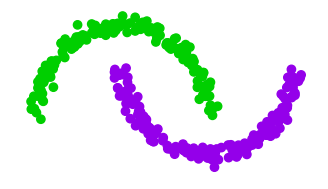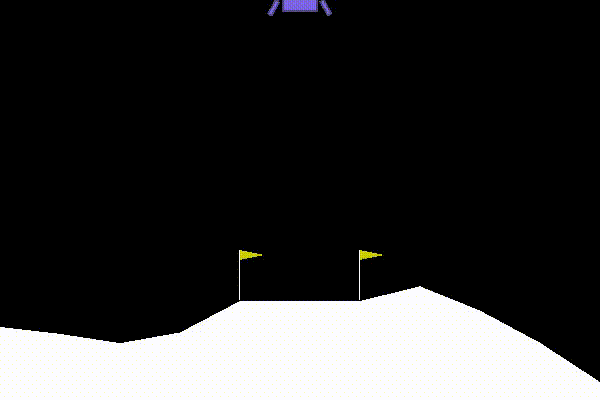Quickstart¶

This section provides with the simplest API to use prevision-quantum-nn and to get started quickly. The easiest way to get started is to use prevision-quantum-nn Applications.

Prevision-quantum-nn allows you to solve two different kind of applications:

Supervised learning
• Classification
• Multi-classification
• RegressionReinforcement learning
• Games
• Yield management
• Autonomous drivingTip

In order to know the capabilities of the models, try the examples stored in the prevision-quantum-nn/examples folder!

First, import the library:

import prevision_quantum_nn as qnn

In order to feed the application, you will first need to encode your problem!

Encoding the problem¶

Refer to the supervised learning section or to the reinforcement learning one depending on the problem you want to solve.

Supervised learning problems¶

In supervised learning tasks, you usually have a dataset encoded either into a numpy array or into a panda DataFrame. Prevision-quantum-nn provides with a simple interface to get started with your data: building a DataSet.

From a numpy array

dataset = qnn.get_dataset_from_numpy(train_features,
train_labels,
val_features=val_features,
val_labels=val_labels)

From pandas DataFrames

dataset = qnn.get_dataset_from_pandas(train_df,
target_columns,
val_df=val_df)

Reinforcement learning problems¶

Reinforcement learning problems must be encoded with the same architecture as the gym framework.

For example, load the Lunar Lander game:

import gym
environment = gym.make("LunarLander-v2")

Building an application¶

Now that the problem has been encoded into a prevision-quantum-nn friendly format, you are able to create an application.

Then, call the API with the name of the application you wish to solve. Applications can be of 4 different kinds:

1. "classification"

2. "multiclassification"

3. "regression"

4. "reinforcement_learning"

For example, for a classification problem, run:

application = qnn.get_application("classification")

Solving the problem¶

Note

The default behavior of an application is the following:

• a qubit architecture will be used, with angle encoding.

• The depth of the circuit is 3 layers.

• The simulation interface is tensorflow.

• Verbosity is activated.

• An early stopper will stop the calculation if the validation loss is not improving anymore.

• The model parameters and weights will be saved at termination.

• The prefix of the output files is “qnn”.

In the case of a supervised learning task, use:

application.solve(dataset)

In the case of reinforcement learning problem, use:

application.solve(environment)

In order to get more control on you applications, visit the advanced section.

Get into production¶

For supervised learning tasks, build new_features and run predict on the model!

prediction = application.model.predict(new_features)

For a reinforcement learning task, build a state and predict the action to take!

action = application.model.get_action(state)

Tip